Thermodynamics and Propulsion

# 8.2 Work and Heat Transfer with Two-Phase Media

We examine the work and heat transfer in quasi-static processes with two-phase systems. For definiteness, consider the system to be a liquid-vapor mixture in a container whose volume can be varied through movement of a piston, as shown in Figure 8.6. The system is kept at constant temperature through contact with a heat reservoir at temperature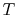. The pressure is thus also constant, but the volume,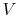, can change. For a fixed mass, the volume is proportional to the specific volume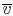so that point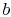in Figure 8.6 must move to the left or the right aschanges. This implies that the amount of mass in each of the two phases, and hence the quality, also changes because mass is transferred from one phase to the other. We wish to find the heat and work transfer associated with the change in mass in each phase. The change in volume can be related to the changes in mass in the two phases as,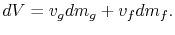The system mass is constant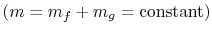so that for any changes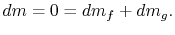We can define the quantity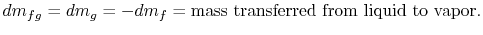In terms of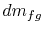the volume change of the system is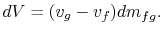The work done is given by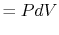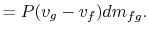The change in internal energy,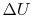, can be found as follows. The internal energy of the system can be expressed in terms of the mass in each phase and the specific internal energy (internal energy per unit mass,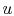) of the phase as,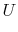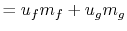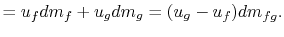Note that the specific internal energy of the two-phase system can be expressed in a similar way as the specific volume in terms of the quality and the specific internal energy of each phase: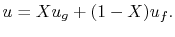Writing the first law for this process: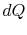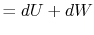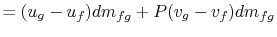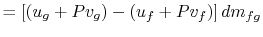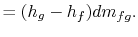The heat needed for the transfer of mass is proportional to the difference in specific enthalpy between vapor and liquid. The pressure and temperature are constant, so that the specific internal energy and the specific enthalpy for the liquid phase and the gas phase are also constant. For a finite change in mass from liquid to vapor,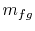, therefore, the quantity of heat needed is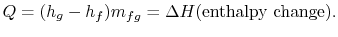The heat needed per unit mass,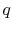, for transformation between the two phases is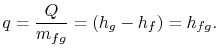The notation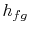refers to the specific enthalpy change between the liquid state and the vapor state. The expression for the amount of heat needed,, is a particular case of the general result that in any reversible process at constant pressure, the heat flowing into, or out of, the system is equal to the enthalpy change. Heat is absorbed if the change is from solid to liquid (heat of fusion), liquid to vapor (heat of vaporization), or solid to vapor (heat of sublimation).

A numerical example is furnished by the vaporization of water at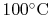:

1. How much heat is needed per unit mass of fluid vaporized?
2. How much work is done per unit mass of fluid vaporized?
3. What is the change in internal energy per unit mass of fluid vaporized?

In addressing these questions, we make use of the fact that problems involving heat and work exchanges in two-phase media are important enough that the values of the specific thermodynamic properties that characterize these transformations have been computed for many different working fluids. The values are given in SB&VW in Tables B.1.1 and B.1.2 for water at saturated conditions and in Tables B.1.3, B.1.4, and B.1.5 for other conditions, as well as for other working fluids, as well as in the Appendix. From these, for water:

• At, the vapor pressure is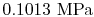.
• The specific enthalpy of the vapor,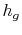, is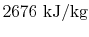and the specific enthalpy of the liquid,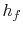, is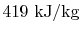.
• The difference in enthalpy between liquid and vapor,, occurs often enough so that it is tabulated also. This is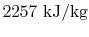.
• The specific volume of the vapor is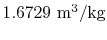and the specific volume of the liquid is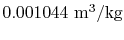.

The heat input to the system is the change in enthalpy between liquid and vapor,, and is equal to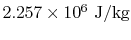.

The work done is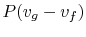which has a value of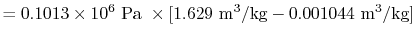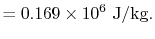The change in internal energy per unit mass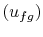can be found from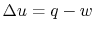or from the tabulated values as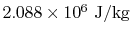. This is much larger than the work done. Most of the heat input is used to change the internal energy rather than appearing as work.

Muddy Points

For the vapor dome, is there vapor and liquid inside the dome and outside is it just liquid or just gas? Is it interchangeable? Is it true for the plasma phase? (MP 8.1)

What is? How do we find it? (MP 8.2)

Reasoning behind the slopes for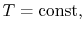lines in the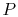-diagram. (MP 8.3)

For a constant pressure heat addition, why is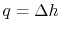? (MP 8.4)

What is latent heat? (MP 8.5)

Why is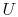a function of? (MP 8.6)

UnifiedTP